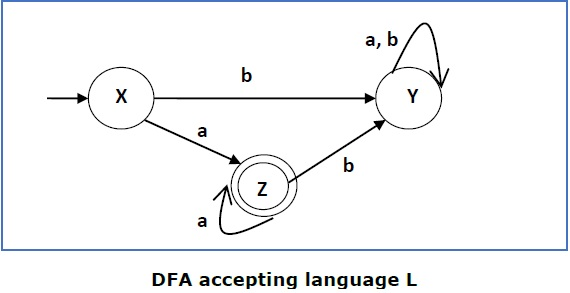# DFA Complement

If (Q, ∑, δ, q0, F) be a DFA that accepts a language L, then the complement of the DFA can be obtained by swapping its accepting states with its non-accepting states and vice versa.

We will take an example and elaborate this below −This DFA accepts the language

L = {a, aa, aaa , ............. }

over the alphabet

∑ = {a, b}

So, RE = a+.

Now we will swap its accepting states with its non-accepting states and vice versa and will get the following −This DFA accepts the language

Ľ = {ε, b, ab ,bb,ba, ............... }

over the alphabet

∑ = {a, b}

Note − If we want to complement an NFA, we have to first convert it to DFA and then have to swap states as in the previous method.